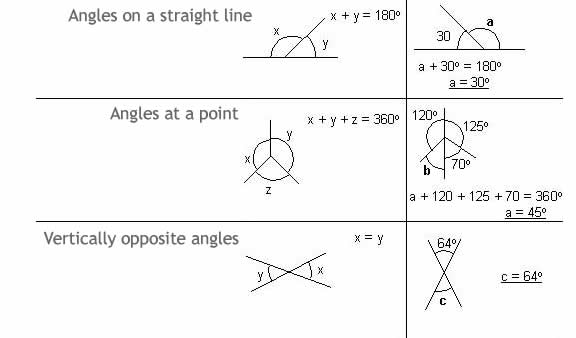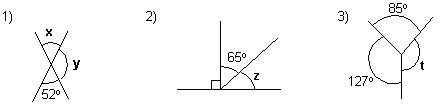# Angles & Straight Lines Math Review

math tutorials > geometry of angles & straight lines

## Angles on a straight line, at a point & vertically opposite angles

Angles on a straight line
The sum of the angles on a straight line is 180º

Angles at a point
The sum of the angles formed at a point is 360º

Vertically opposite angles
When 2 straight lines intersect, vertically opposite angles are formed. These angles are equal.## Geometry problems for you to try.Solutions

x = 52º

y = 128º

z = 25º

t = 148º